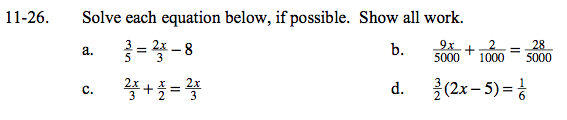### Home > GC > Chapter 11 > Lesson 11.1.2 > Problem11-26

11-26.Multiply both sides of the equation by the lowest common denominator.

$\left(\frac{3}{5}=\frac{2x}{3}-8\right)\cdot 15$

3 · 3 = 2x · 5 − 8 · 15

9 = 10x − 120

129 = 10x

12.9 = x

Instead of removing fractions altogether, you may find it simplest to convert the second fraction so that it has the same denominator as the others. The denominator can be changed by multiplying the numerator and denominator by the same number.

Multiply by 6 and then distribute.

$x=\frac{23}{9} \approx 2.56$